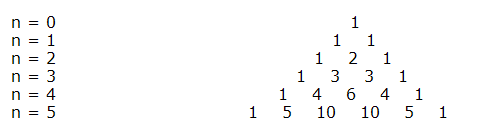# Binomial Expansion

Binomial

A binomial is an expression that contains two terms.

Example: (a + b), (1 - a),k - h),(1 + x)2,(3x + 1)4.

The expansion of (1 + x)3 can be easily found:
(1 + x)3
= (1 + x)2(1 + x)
= (1 + 2x + x2) (1 + x)
= 1 + 2x + x2 + x + 2x2 + x3
= 1 + 3x + 3x2 + x3
Similarly, we can use this method to find (1 + x)4, (1 + x)5 and (1 + x)6.

Look at the following expansions of (1 + x)n:
(1 + x)0 = 1
(1 + x)1 = 1 + x
(1 + x)2 = 1 + 2x + x2
(1 + x)3 = 1 + 3x + 3x2 + x3
(1 + x)4 = 1 + 4x + 6x2 + 4x3 + x4
(1 + x)5 = 1 + 5x + 10x2 + 10x3 + 5x4 + x5

List the coefficients in a triangular array:This array is known as the Pascal's Triangle.